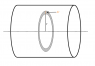# Ampere's Law: Cylindrical conducter with varying current

• Renaldo

## Homework Statement

The current density of a cylindrical conductor of radius R varies as J(r) = J0e−r/R (in the region from zero to R). Express the magnitude of the magnetic field in the regions r < R and r > R. (Use any variable or symbol stated above along with the following as necessary: μ0.)

Produce a sketch of the radial dependence, B(r).

## Homework Equations

Ampere's Law

$\oint B \bullet ds$ = μ0ienc

## The Attempt at a Solution

At r < R:

B = μ0J(r)A/2∏r

J(r) = J0e−r/R
J(r)A = J0e−r/R(∏r2)

B = (μ0J0e−r/Rr)/2

This is not correct.

How current is defined in terms of current density?

(Hint: The relation between current and current density involves a integral.)

I = J(r)A
dI = J0∏r2e-r/Rdr

I = J0∏∫r2e-r/Rdr

I used a computer to solve the integral, which is an integration by parts.

I = -J0∏R(r2+2rR+2R2)/er/R

B = μ0I/2∏r

B = -μ0J0R(r2+2rR+2R2)/2rer/R

I = J(r)A

You can't write that. According to the definition,
$$I=\int \vec{j}\cdot\vec{dA}$$

What is dA?

In that definition, is J constant? It seems to me that it would not be, but I don't know how to integrate

∫J0e-r/Rda

In that definition, is J constant? It seems to me that it would not be, but I don't know how to integrate

∫J0e-r/Rda

No, J is not constant. You have the expression for J and it varies with r. About the differential area dA, see the attachment. We have to find the total current enclosed within the Amperian loop. As we move out away from the axis of cylinder, the current density varies so we select a very small area (differential area, dA) where we can assume that current density is effectively constant. Then we sum up (or integrate) the expression we get for the current passing through that small region. See the attachment. Can you calculate dA now? I have drawn two circles of radius r and the other with r+dr. The shaded region is dA.

#### Attachments

•cylinder.png
7 KB · Views: 408
da = 2∏rdr

2∏J0∫e(-r/R)rdr = -2J0∏R(r+R)/er/R

B = -μ0J0R(r+R)/rer/R

That's what I get and it isn't correct.

Last edited:
What were your limits? Do you know how to evaluate a definite integral?

limits of integration were from 0 to r, r < R.

limits of integration were from 0 to r, r < R.

Right but check your work again. I get a different answer. (##e^0=1##)